# Solid Elliptical Section Civil Engineering (CE) Notes | EduRev

## Civil Engineering (CE) : Solid Elliptical Section Civil Engineering (CE) Notes | EduRev

The document Solid Elliptical Section Civil Engineering (CE) Notes | EduRev is a part of the Civil Engineering (CE) Course Advanced Solid Mechanics - Notes, Videos, MCQs & PPTs.
All you need of Civil Engineering (CE) at this link: Civil Engineering (CE)

Solid elliptical section

The ﬁrst cross section shape that we consider is that of an ellipse. That is we study a bar with elliptical cross section, whose boundary is deﬁned by the function,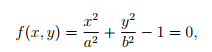(9.58)

where a and b are constants and we have assumed that the major and minor axis of the ellipse coincides with the coordinate basis, as shown in the ﬁgure 9.11.

Choosing the Prandtl stress function to be,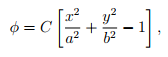(9.59)

where C is a constant, we ﬁnd that it satisﬁes the boundary condition (9.50).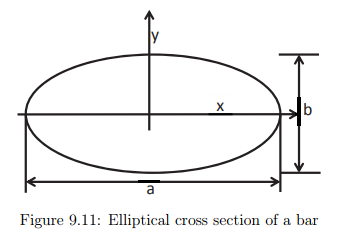Substituting the stress function, (9.59) in the equation (9.47) we obtain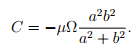(9.60)

Using (9.59) and (9.60) in (9.57), twisting moment is obtained as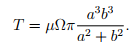(9.61)

Corresponding to the stress function (9.59) the shear stresses are computed to be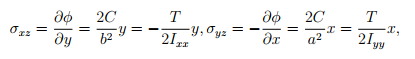(9.62)

where Ixx = πab3/4, Iyy = ba3/4 and we have substituted for the constant, C from equation (9.60). The variation of these shear stresses over the cross section is indicated in ﬁgure 9.12.

The resultant shear stress in the xy plane is given by,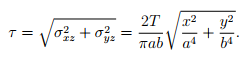(9.63)

It is clear from equation (9.63) that the extremum shear stress occurs at (0,0) or the boundary of the cross section. It can be seen that at (0,0) minimum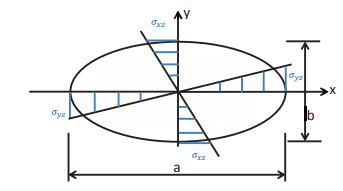Figure 9.12: Shear stresses in a bar with elliptical cross section subjected to end torsion

shear stress occurs, as τ = 0 at this location. At the boundary the extremum shear stresses would occur at (0,±b) and (±a,0). Thus, the extremum shear stresses are τext = 2T/(πab2) at (0,±b) and τext = 2T/(πba2) at (±a,0). If a > b, then the maximum shear stress in the elliptical cross section is,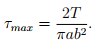(9.64)

Substituting (9.59) in equations (9.45) and (9.46) and rearranging we obtain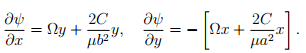(9.65)

Solving the diﬀerential equations (9.65) we obtain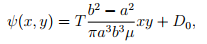(9.66)

where D0 is a constant. Since, there is no rigid body translation, we require that ψ(0,0) = 0. Hence, D0 = 0. Thus, we ﬁnd that the section warps into a diagonally symmetric surface as shown in ﬁgure 9.13.

Rearranging the equation (9.61) we can get Ω as a function of torque, T
as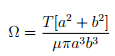(9.67)

The entire displacement ﬁeld, (9.33) for a bar with elliptical cross section is now known that we have found the warping function (9.66). The deformation of a bar with the elliptical cross section computed using this displacement ﬁeld is shown in ﬁgure 9.14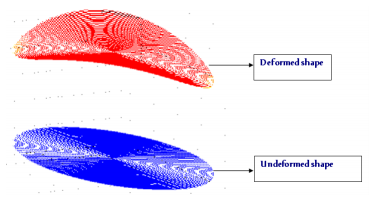Figure 9.13: Warping deformation of a elliptical cross section due to end torsion

Before concluding this section let us see the error that would have been made if one analyzes the elliptical section as a closed section. The polar moment of inertia for the elliptical section could be computed and shown to be J = πab(a2 + b2)/4. Then, the angle of twist per unit length computed from (9.17) would be,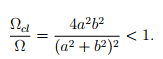(9.68)

The ratio of Ωcl/Ω, computed from equations (9.68) and (9.67) is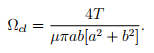(9.69)

Thus, for a given torque open section twists more. Next, we examine the shear stresses. If one uses (9.17) to compute the shear stresses, they would erroneously conclude that the maximum shear stress occurs at (±a,0), by virtue of these points being farthest from the center of twist and the value of this maximum shear stress would be,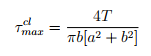(9.70)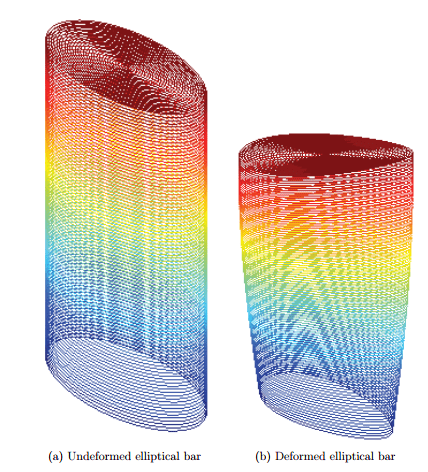Figure 9.14: A bar with elliptical cross section subjected to end twisting moment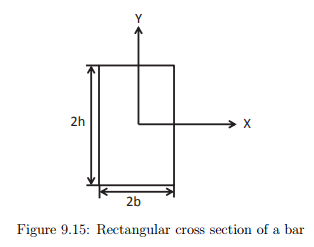Comparing equations (9.64) and (9.70) we ﬁnd that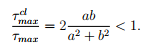(9.71)

Thus, closed section underestimates the stresses in the bar.

Hence, from both strength and serviceability point of view assuming the elliptical cross section to be closed would result in an unsafe design.

Offer running on EduRev: Apply code STAYHOME200 to get INR 200 off on our premium plan EduRev Infinity!

## Advanced Solid Mechanics - Notes, Videos, MCQs & PPTs

42 videos|61 docs

,

,

,

,

,

,

,

,

,

,

,

,

,

,

,

,

,

,

,

,

,

;